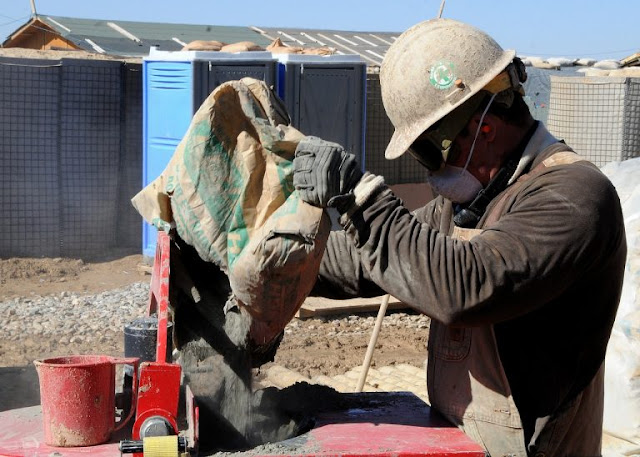Concrete Mix Design

# Concrete Mix DesignConcrete Mix Design (IS 10262 : 2009)
For proper concrete mix design, the following thirteen (13) points of data are required:
1. Type of cement;
3. Minimum cement content;
4. Maximum cement content;
5. Maximum water-cement ratio;
6. Maximum nominal size of aggregate;
7. Workability;
8. Exposure conditions (Table 4 and Table 5 of IS -456);
9. The maximum temperature of concrete at the time of placing;
10. Method of transporting and placing;
11. Early age strength requirements (if necessary):
12. Type of aggregate;
13. Whether an admixture shall or shall not be used, the type of admixture, and the use condition.
Procedure for concrete mix design requires following seven (7) step by step process:
1. Target strength of concrete calculation
2. Water-cement ratio selection
3. Selection of water content
4. Selection of cement content
5. Aggregate ratio calculation
6. Calculation of aggregate content
7. Trial mixes to test strength of concrete mix design

### I. Target Strength of Concrete Calculation

Target strength is denoted by ft. Target strength is obtained by adding the characteristic compressive strength of concrete at 28 days denoted by fck and value of standard deviation denoted by (s).
f= fck + 1.65 s
The standard deviation (s) can be found by using the table below based on the grade of concrete.
 Grade of concrete Standard deviation (N/mm2) M10 3.5 M15 3.5 M20 4.0 M25 4.0 M30 5.0 M35 5.0 M40 5.0 M45 5.0 M50 5.0

### II: Water-Cement Ratio Selection

Water-cement ratio is the ratio of the weight of water to the weight of the cement in the concrete mix. This is a very important part of concrete mix design in order to make the concrete feasible. From the below curve, the water-cement ratio is selected for twenty-eight (28) days characteristic compressive strength of concrete.
Ratio of the weight of water to weight of cement in the concrete mix is water-cement ratio. It is the important consideration in concrete mix design to make the concrete workable. Water cement ratio is selected from the below curve for 28 days characteristic compressive strength of concrete.
Graph: Water-Cement Ratio Selection for Concrete Mix Design
In a similar fashion, we can determine the water-cement ratio from the 7-day concrete strength. You can observe this in the graph below. The curves (A through F) are determined on the basis of the strength from the water-cement ratio.
Graph: Concrete Compressive Strength vs. Water-Cement Ratio

### III: Selection of Water Content

In the below table, select the water content to get required workability with the help of nominal maximum size of aggregate. Use the table below only when angular shaped aggregates are used in the concrete. Furthermore, the slump should be between 25 and 50mm.
 Nominal Maximum Size of Aggregate Maximum Water Content 10mm 208 20mm 186 40mm 165
The adjustments below must be made if the shape of aggregate or slump value differs from the above table.
 If This Condition Make This Adjustment Sub-Angular Aggregate Reduce the selected value by 10% Crushed Stone Gravel Reduce the selected value by 20kg Rounded Gravel Reduce the selected value by 25kg Plasticizer Used Decrease the selected value by 5-10% Superplasticizer Used Decrease the selected value by 20-30% For Each 25mm of Slump Increment Increase the selected value by 3%

### IV: Selection of Cement Content

In Step II, we determined the water-cement ratio. The quantity of water is determined in Step III. With these two conditions, we can now easily calculate the quantity of cement for concrete. However, the value must satisfy the minimum conditions set forth in the below table.
##### Cement Content for Reinforced Concrete
 Exposure Reinforced Cement Concrete (RCC) Minimum Cement Content in Kg/m3 Maximum Free Water-Cement Ratio Minimum Grade of Concrete Mild 300 0.55 M20 Moderate 300 0.5 M25 Severe 320 0.45 M30 Very severe 340 0.45 M35 Extreme 360 0.4 M40

### V. Aggregate Ratio Calculation

Using the nominal maximum size of aggregate, we can calculate the ratio of volume of coarse aggregate and volume of total aggregate for different zones of fine aggregate. Use the table below to do so.
 Nominal maximum size of aggregate Ratio of volume of coarse aggregate and volume of total aggregate for different zones of fine aggregate Zone 1 Zone 2 Zone 3 Zone 4 10mm 0.44 0.46 0.48 0.50 20mm 0.6 0.62 0.64 0.66 40mm 0.69 0.71 0.73 0.75

### VI: Calculation of Aggregate Content

We have now determined the coarse aggregate volume ratio in the total aggregate volume. Using the volume of coarse aggregate will can easily learn the volume of fine aggregate.
The following formulae can be used to find the volume of fine and coarse aggregates.

The Formula for Mass of Fine Aggregate:The Formula for the Mass of Coarse Aggregate:Using the following variables:
V = volume of concrete
W = water content
C = cement content
Gc = sp. Gravity of cement
P = aggregate ration obtained in step VI
FA & CA = Masses of Fine Aggregates and Coarse Aggregates

### VII: Trial Mixes to Test Strength of Concrete Mix Design

Complete a trial test by making at least three 150mm sized cubes based on the values achieved from the previous steps. Test the three cubes to determine whether the required strength was achieved. Make the proper adjustments if the strength needed was not correct.